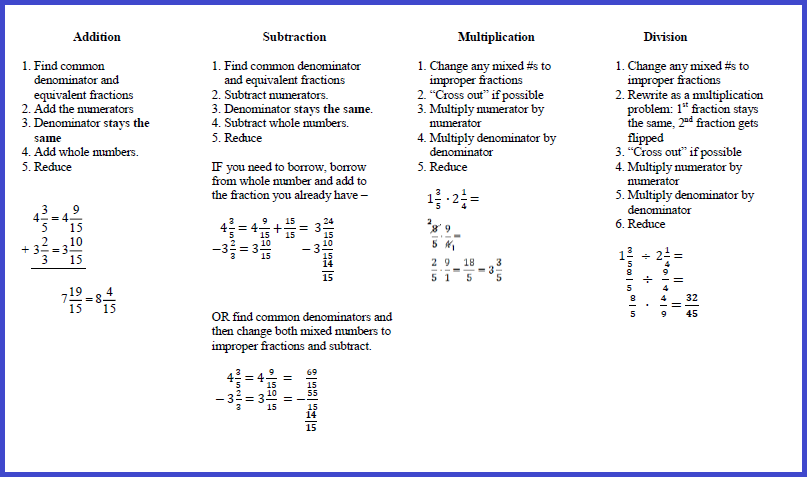## Monday

### Multiplying Mixed Numbers

We've been working on adding, subtracting and multiplying mixed numbers, and with all the snow days and delays, instruction and practice have been chopped up. Students learned the process to multiply mixed numbers on Friday: change them to improper fractions, and then multiply the numerators and the denominators. Since we haven't spent much time on this yet, and because I know they often get confused when they move from adding and subtracting to multiplying (common denominator...no common denominator...what do I do??), I made them a fraction operations "note sheet" with the steps that need to be followed for each one.Click to download

After reviewing the steps to multiply mixed numbers and practicing a few problems together, the students started practicing on their own (the review and practice together was quick since there was a two-hour delay today and the period was only 20 minutes long!). Most students were ok, but then I found one who decided to multiply the whole numbers together and then multiply the fractions, so 5_1/2 x 3_3/5 = 15_3/10.  This gave me the opportunity for a very brief discussion about WHY we need to change those mixed numbers to improper fractions.

My next two classes were longer, so I started these classes by asking the students to solve the problem 2_3/8 x 3 5/7. Some gave the answer of 6_15/56 and others came up with 8_23/28.  I told them which answer was correct, and asked them to prove to me WHY it was correct.  Most students responded that it was correct because if they did it the "improper fraction way," that's the answer they got. I told them that wasn't enough, because how do they know that the "improper fraction way" was really correct.....just because I said so?  I asked what the improper fraction way does that the other way (multiplying whole numbers and then fractions) doesn't.  In the first class, a couple of students figured it out right away - the improper fractions include both the whole number and the fraction, so all parts of the mixed number are used, but the other way misses some parts.  The second class took a little longer, and a few more probing questions, but finally one student figured it out.

We went through the steps we would need to take if we didn't use improper fractions:

After all of this work, students agreed that they would much rather use improper fractions to multiply mixed numbers! AND they now understand WHY using improper fractions works.

I can't wait to start dividing fractions!